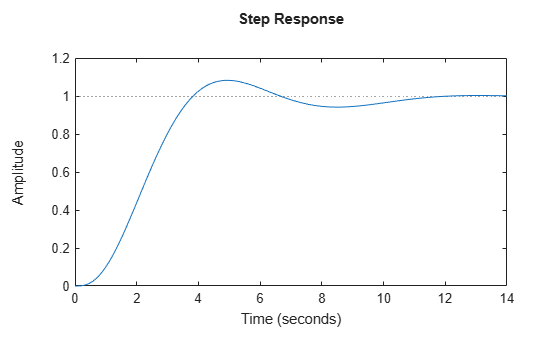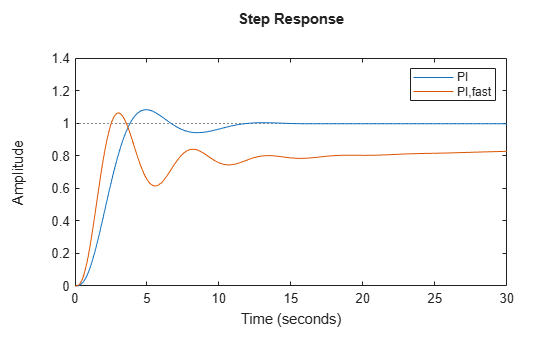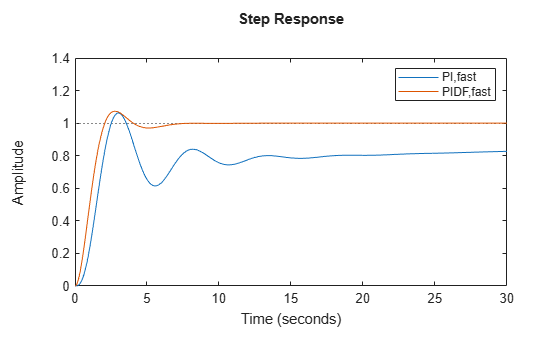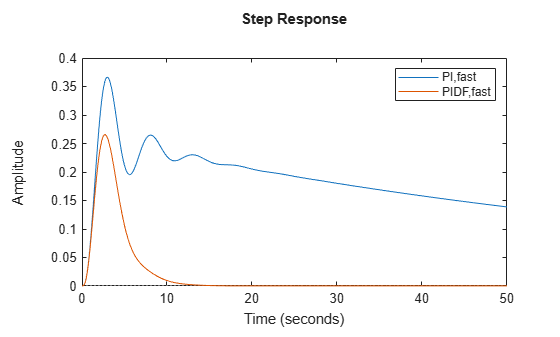Documentation

## PID Controller Design at the Command Line

This example shows how to design a PID controller for the plant given by:

`$sys=\frac{1}{{\left(s+1\right)}^{3}}.$`

As a first pass, create a model of the plant and design a simple PI controller for it.

```sys = zpk([],[-1 -1 -1],1); [C_pi,info] = pidtune(sys,'PI')```
```C_pi = 1 Kp + Ki * --- s with Kp = 1.14, Ki = 0.454 Continuous-time PI controller in parallel form. ```
```info = struct with fields: Stable: 1 CrossoverFrequency: 0.5205 PhaseMargin: 60.0000 ```

`C_pi` is a `pid` controller object that represents a PI controller. The fields of `info` show that the tuning algorithm chooses an open-loop crossover frequency of about 0.52 rad/s.

Examine the closed-loop step response (reference tracking) of the controlled system.

```T_pi = feedback(C_pi*sys, 1); step(T_pi)```To improve the response time, you can set a higher target crossover frequency than the result that `pidtune` automatically selects, 0.52. Increase the crossover frequency to 1.0.

`[C_pi_fast,info] = pidtune(sys,'PI',1.0)`
```C_pi_fast = 1 Kp + Ki * --- s with Kp = 2.83, Ki = 0.0495 Continuous-time PI controller in parallel form. ```
```info = struct with fields: Stable: 1 CrossoverFrequency: 1 PhaseMargin: 43.9973 ```

The new controller achieves the higher crossover frequency, but at the cost of a reduced phase margin.

Compare the closed-loop step response with the two controllers.

```T_pi_fast = feedback(C_pi_fast*sys,1); step(T_pi,T_pi_fast) axis([0 30 0 1.4]) legend('PI','PI,fast')```This reduction in performance results because the PI controller does not have enough degrees of freedom to achieve a good phase margin at a crossover frequency of 1.0 rad/s. Adding a derivative action improves the response.

Design a PIDF controller for `Gc` with the target crossover frequency of 1.0 rad/s.

`[C_pidf_fast,info] = pidtune(sys,'PIDF',1.0)`
```C_pidf_fast = 1 s Kp + Ki * --- + Kd * -------- s Tf*s+1 with Kp = 2.72, Ki = 0.985, Kd = 1.72, Tf = 0.00875 Continuous-time PIDF controller in parallel form. ```
```info = struct with fields: Stable: 1 CrossoverFrequency: 1 PhaseMargin: 60.0000 ```

The fields of info show that the derivative action in the controller allows the tuning algorithm to design a more aggressive controller that achieves the target crossover frequency with a good phase margin.

Compare the closed-loop step response and disturbance rejection for the fast PI and PIDF controllers.

```T_pidf_fast = feedback(C_pidf_fast*sys,1); step(T_pi_fast, T_pidf_fast); axis([0 30 0 1.4]); legend('PI,fast','PIDF,fast');```You can compare the input (load) disturbance rejection of the controlled system with the fast PI and PIDF controllers. To do so, plot the response of the closed-loop transfer function from the plant input to the plant output.

```S_pi_fast = feedback(sys,C_pi_fast); S_pidf_fast = feedback(sys,C_pidf_fast); step(S_pi_fast,S_pidf_fast); axis([0 50 0 0.4]); legend('PI,fast','PIDF,fast');```This plot shows that the PIDF controller also provides faster disturbance rejection.